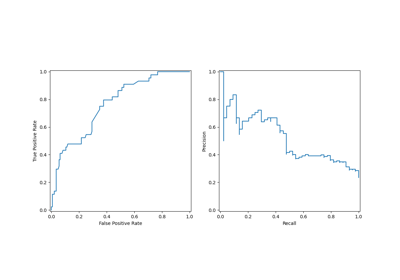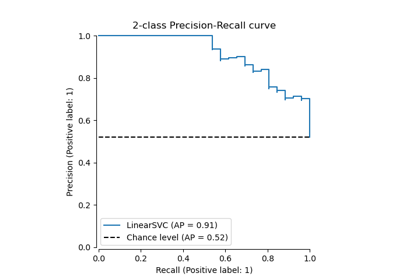# sklearn.metrics.PrecisionRecallDisplay¶

class sklearn.metrics.PrecisionRecallDisplay(precision, recall, *, average_precision=None, estimator_name=None, pos_label=None, prevalence_pos_label=None)[source]

Precision Recall visualization.

It is recommend to use from_estimator or from_predictions to create a PrecisionRecallDisplay. All parameters are stored as attributes.

Read more in the User Guide.

Parameters:
precisionndarray

Precision values.

recallndarray

Recall values.

average_precisionfloat, default=None

Average precision. If None, the average precision is not shown.

estimator_namestr, default=None

Name of estimator. If None, then the estimator name is not shown.

pos_labelint, float, bool or str, default=None

The class considered as the positive class. If None, the class will not be shown in the legend.

New in version 0.24.

prevalence_pos_labelfloat, default=None

The prevalence of the positive label. It is used for plotting the chance level line. If None, the chance level line will not be plotted even if plot_chance_level is set to True when plotting.

New in version 1.3.

Attributes:
line_matplotlib Artist

Precision recall curve.

chance_level_matplotlib Artist or None

The chance level line. It is None if the chance level is not plotted.

New in version 1.3.

ax_matplotlib Axes

Axes with precision recall curve.

figure_matplotlib Figure

Figure containing the curve.

precision_recall_curve

Compute precision-recall pairs for different probability thresholds.

PrecisionRecallDisplay.from_estimator

Plot Precision Recall Curve given a binary classifier.

PrecisionRecallDisplay.from_predictions

Plot Precision Recall Curve using predictions from a binary classifier.

Notes

The average precision (cf. average_precision_score) in scikit-learn is computed without any interpolation. To be consistent with this metric, the precision-recall curve is plotted without any interpolation as well (step-wise style).

You can change this style by passing the keyword argument drawstyle="default" in plot, from_estimator, or from_predictions. However, the curve will not be strictly consistent with the reported average precision.

Examples

>>> import matplotlib.pyplot as plt
>>> from sklearn.datasets import make_classification
>>> from sklearn.metrics import (precision_recall_curve,
...                              PrecisionRecallDisplay)
>>> from sklearn.model_selection import train_test_split
>>> from sklearn.svm import SVC
>>> X, y = make_classification(random_state=0)
>>> X_train, X_test, y_train, y_test = train_test_split(X, y,
...                                                     random_state=0)
>>> clf = SVC(random_state=0)
>>> clf.fit(X_train, y_train)
SVC(random_state=0)
>>> predictions = clf.predict(X_test)
>>> precision, recall, _ = precision_recall_curve(y_test, predictions)
>>> disp = PrecisionRecallDisplay(precision=precision, recall=recall)
>>> disp.plot()
<...>
>>> plt.show()


Methods

 from_estimator(estimator, X, y, *[, ...]) Plot precision-recall curve given an estimator and some data. from_predictions(y_true, y_pred, *[, ...]) Plot precision-recall curve given binary class predictions. plot([ax, name, plot_chance_level, ...]) Plot visualization.
classmethod from_estimator(estimator, X, y, *, sample_weight=None, pos_label=None, drop_intermediate=False, response_method='auto', name=None, ax=None, plot_chance_level=False, chance_level_kw=None, **kwargs)[source]

Plot precision-recall curve given an estimator and some data.

Parameters:
estimatorestimator instance

Fitted classifier or a fitted Pipeline in which the last estimator is a classifier.

X{array-like, sparse matrix} of shape (n_samples, n_features)

Input values.

yarray-like of shape (n_samples,)

Target values.

sample_weightarray-like of shape (n_samples,), default=None

Sample weights.

pos_labelint, float, bool or str, default=None

The class considered as the positive class when computing the precision and recall metrics. By default, estimators.classes_ is considered as the positive class.

drop_intermediatebool, default=False

Whether to drop some suboptimal thresholds which would not appear on a plotted precision-recall curve. This is useful in order to create lighter precision-recall curves.

New in version 1.3.

response_method{‘predict_proba’, ‘decision_function’, ‘auto’}, default=’auto’

Specifies whether to use predict_proba or decision_function as the target response. If set to ‘auto’, predict_proba is tried first and if it does not exist decision_function is tried next.

namestr, default=None

Name for labeling curve. If None, no name is used.

axmatplotlib axes, default=None

Axes object to plot on. If None, a new figure and axes is created.

plot_chance_levelbool, default=False

Whether to plot the chance level. The chance level is the prevalence of the positive label computed from the data passed during from_estimator or from_predictions call.

New in version 1.3.

chance_level_kwdict, default=None

Keyword arguments to be passed to matplotlib’s plot for rendering the chance level line.

New in version 1.3.

**kwargsdict

Keyword arguments to be passed to matplotlib’s plot.

Returns:
displayPrecisionRecallDisplay

PrecisionRecallDisplay.from_predictions

Plot precision-recall curve using estimated probabilities or output of decision function.

Notes

The average precision (cf. average_precision_score) in scikit-learn is computed without any interpolation. To be consistent with this metric, the precision-recall curve is plotted without any interpolation as well (step-wise style).

You can change this style by passing the keyword argument drawstyle="default". However, the curve will not be strictly consistent with the reported average precision.

Examples

>>> import matplotlib.pyplot as plt
>>> from sklearn.datasets import make_classification
>>> from sklearn.metrics import PrecisionRecallDisplay
>>> from sklearn.model_selection import train_test_split
>>> from sklearn.linear_model import LogisticRegression
>>> X, y = make_classification(random_state=0)
>>> X_train, X_test, y_train, y_test = train_test_split(
...         X, y, random_state=0)
>>> clf = LogisticRegression()
>>> clf.fit(X_train, y_train)
LogisticRegression()
>>> PrecisionRecallDisplay.from_estimator(
...    clf, X_test, y_test)
<...>
>>> plt.show()

classmethod from_predictions(y_true, y_pred, *, sample_weight=None, pos_label=None, drop_intermediate=False, name=None, ax=None, plot_chance_level=False, chance_level_kw=None, **kwargs)[source]

Plot precision-recall curve given binary class predictions.

Parameters:
y_truearray-like of shape (n_samples,)

True binary labels.

y_predarray-like of shape (n_samples,)

Estimated probabilities or output of decision function.

sample_weightarray-like of shape (n_samples,), default=None

Sample weights.

pos_labelint, float, bool or str, default=None

The class considered as the positive class when computing the precision and recall metrics.

drop_intermediatebool, default=False

Whether to drop some suboptimal thresholds which would not appear on a plotted precision-recall curve. This is useful in order to create lighter precision-recall curves.

New in version 1.3.

namestr, default=None

Name for labeling curve. If None, name will be set to "Classifier".

axmatplotlib axes, default=None

Axes object to plot on. If None, a new figure and axes is created.

plot_chance_levelbool, default=False

Whether to plot the chance level. The chance level is the prevalence of the positive label computed from the data passed during from_estimator or from_predictions call.

New in version 1.3.

chance_level_kwdict, default=None

Keyword arguments to be passed to matplotlib’s plot for rendering the chance level line.

New in version 1.3.

**kwargsdict

Keyword arguments to be passed to matplotlib’s plot.

Returns:
displayPrecisionRecallDisplay

PrecisionRecallDisplay.from_estimator

Plot precision-recall curve using an estimator.

Notes

The average precision (cf. average_precision_score) in scikit-learn is computed without any interpolation. To be consistent with this metric, the precision-recall curve is plotted without any interpolation as well (step-wise style).

You can change this style by passing the keyword argument drawstyle="default". However, the curve will not be strictly consistent with the reported average precision.

Examples

>>> import matplotlib.pyplot as plt
>>> from sklearn.datasets import make_classification
>>> from sklearn.metrics import PrecisionRecallDisplay
>>> from sklearn.model_selection import train_test_split
>>> from sklearn.linear_model import LogisticRegression
>>> X, y = make_classification(random_state=0)
>>> X_train, X_test, y_train, y_test = train_test_split(
...         X, y, random_state=0)
>>> clf = LogisticRegression()
>>> clf.fit(X_train, y_train)
LogisticRegression()
>>> y_pred = clf.predict_proba(X_test)[:, 1]
>>> PrecisionRecallDisplay.from_predictions(
...    y_test, y_pred)
<...>
>>> plt.show()

plot(ax=None, *, name=None, plot_chance_level=False, chance_level_kw=None, **kwargs)[source]

Plot visualization.

Extra keyword arguments will be passed to matplotlib’s plot.

Parameters:
axMatplotlib Axes, default=None

Axes object to plot on. If None, a new figure and axes is created.

namestr, default=None

Name of precision recall curve for labeling. If None, use estimator_name if not None, otherwise no labeling is shown.

plot_chance_levelbool, default=False

Whether to plot the chance level. The chance level is the prevalence of the positive label computed from the data passed during from_estimator or from_predictions call.

New in version 1.3.

chance_level_kwdict, default=None

Keyword arguments to be passed to matplotlib’s plot for rendering the chance level line.

New in version 1.3.

**kwargsdict

Keyword arguments to be passed to matplotlib’s plot.

Returns:
displayPrecisionRecallDisplay

Object that stores computed values.

Notes

The average precision (cf. average_precision_score) in scikit-learn is computed without any interpolation. To be consistent with this metric, the precision-recall curve is plotted without any interpolation as well (step-wise style).

You can change this style by passing the keyword argument drawstyle="default". However, the curve will not be strictly consistent with the reported average precision.

## Examples using sklearn.metrics.PrecisionRecallDisplay¶Visualizations with Display Objects

Visualizations with Display ObjectsPrecision-Recall

Precision-Recall

## Examples using sklearn.metrics.PrecisionRecallDisplay.from_estimator¶Precision-Recall

Precision-Recall

## Examples using sklearn.metrics.PrecisionRecallDisplay.from_predictions¶Precision-Recall

Precision-Recall